### 14.2.2.3 Resolution completeness for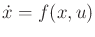Beyond the trivial case of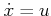, the reachability graph is usually not a simple grid. Even ifis bounded, the reachability graph may have an infinite number of vertices, even though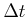is fixed and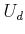is finite. For a simple example, consider the Dubins car under the discretization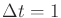. Fix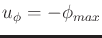(turn left) for all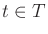. This branch alone generates a countably infinite number of vertices in the reachability graph. The circumference of the circle is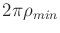, in which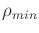is the minimum turning radius. Let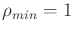. Since the circumference is an irrational number, it is impossible to revisit the initial point by travelingseconds for some integer. It is even impossible to revisit any point on the circle. The set of vertices in the reachability graph is actually dense in the circle. This did not happen in Figure 14.6 becauseand the circumference were rationally related (i.e., one can be obtained from the other via multiplication by a rational number). Consider what happens in the current example when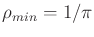and.

Suppose thatand the discrete-time model is used. To ensure convergence of the discrete-time approximation,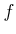must be well-behaved. This can be established by requiring that all of the derivatives ofwith respect toandare bounded above and below by a constant. More generally,is assumed to be Lipschitz, which is an equivalent condition for cases in which the derivatives exist, but it also applies at points that are not differentiable. If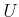is finite, then the Lipschitz condition is that there exists some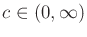such that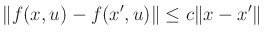(14.10)

for all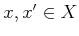, for all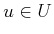, and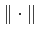denotes a norm on. Ifis infinite, then the condition is that there must exist somesuch that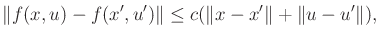(14.11)

for all, and for all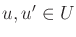. Intuitively, the Lipschitz condition indicates that ifandare approximated by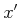and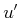, then the error when substituted intowill be manageable. If convergence to optimal trajectories with respect to a cost functional is important, then Lipschitz conditions are also needed for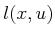. Under such mild assumptions, ifand the dispersion of samples ofis driven down to zero, then the trajectories obtained from integrating discrete action sequences come arbitrarily close to solution trajectories. In other words, action sequences provide arbitrarily close approximations to any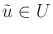. Ifis Lipschitz, then the integration of (14.14) yields approximately the same result for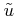as the approximating action sequence.

In the limit asand the dispersion ofapproach zero, the reachability graph becomes dense in the reachable set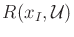. Ensuring a systematic search for the case of a grid was not difficult because there is only a finite number of vertices at each resolution. Unfortunately, the reachability graph may generally have a countably infinite number of vertices for some fixed discrete-time model, even ifis bounded.

To see that resolution-complete algorithms nevertheless exist if the reachability graph is countably infinite, consider triangular enumeration, which proves that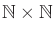is countable, in which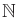is the set of natural numbers. The proof proceeds by giving a sequence that starts at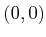and proceeds by sweeping diagonally back and forth across the first quadrant. In the limit, all points are covered. The same idea can be applied to obtain resolution-complete algorithms. A sequence of discrete-time models can be made for which the time stepand the dispersion of the sampling ofapproach zero. Each discretization produces a reachability graph that has a countable number of vertices.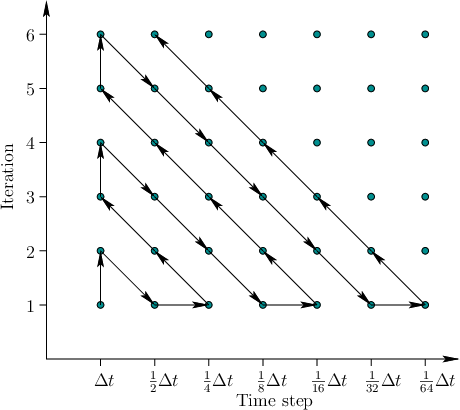A resolution-complete algorithm can be made by performing the same kind of zig-zagging that was used to show thatis countable. See Figure 14.7; suppose thatis finite and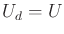. Along the horizontal axis is a sequence of improving discrete-time models. Each model generates its own reachability graph, for which a systematic search eventually explores all of its vertices. Imagine this exploration occurs one step at a time, in which one new vertex is reached in each step. The vertical axis in Figure 14.7 indicates the number of vertices reached so far by the search algorithm. A countably infinite set of computers could explore all of reachability graphs in parallel. With a single computer, it can still be assured that everything is eventually explored by zig-zagging as shown. Thus a resolution-complete algorithm always exists ifis finite. Ifis not finite, thenmust also be refined as the time step is decreased. Of course, there are numerous other ways to systematically explore all of the reachability graphs. The challenging task is to find a way that leads to good performance in practice.

The discussion so far has assumed that a sampling-based algorithm can uncover a subgraph of the reachability graph. This neglects numerical issues such as arithmetic precision and numerical integration error. Such issues can additionally be incorporated into a resolution completeness analysis .

Steven M LaValle 2020-08-14